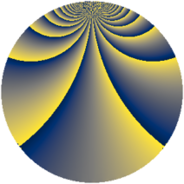# Properties

 Label 930.2.bkLevel $930$ Weight $2$ Character orbit 930.bk Rep. character $\chi_{930}(47,\cdot)$ Character field $\Q(\zeta_{20})$ Dimension $512$ Newform subspaces $1$ Sturm bound $384$ Trace bound $0$

# Related objects

## Defining parameters

 Level: $$N$$ $$=$$ $$930 = 2 \cdot 3 \cdot 5 \cdot 31$$ Weight: $$k$$ $$=$$ $$2$$ Character orbit: $$[\chi]$$ $$=$$ 930.bk (of order $$20$$ and degree $$8$$) Character conductor: $$\operatorname{cond}(\chi)$$ $$=$$ $$465$$ Character field: $$\Q(\zeta_{20})$$ Newform subspaces: $$1$$ Sturm bound: $$384$$ Trace bound: $$0$$

## Dimensions

The following table gives the dimensions of various subspaces of $$M_{2}(930, [\chi])$$.

Total New Old
Modular forms 1600 512 1088
Cusp forms 1472 512 960
Eisenstein series 128 0 128

## Trace form

 $$512q + 8q^{3} - 12q^{7} + O(q^{10})$$ $$512q + 8q^{3} - 12q^{7} - 8q^{10} - 8q^{12} + 8q^{15} + 128q^{16} + 28q^{22} + 32q^{25} + 44q^{27} + 8q^{28} + 24q^{30} + 8q^{31} + 28q^{33} + 176q^{37} + 24q^{42} - 40q^{45} - 24q^{46} + 12q^{48} - 16q^{51} - 40q^{55} + 40q^{57} + 56q^{58} + 12q^{60} - 32q^{61} - 224q^{63} + 32q^{66} + 48q^{67} + 24q^{70} + 40q^{73} - 32q^{75} + 48q^{76} - 76q^{78} - 96q^{81} + 24q^{85} - 80q^{87} - 72q^{88} + 16q^{90} + 80q^{91} - 168q^{93} - 112q^{97} + O(q^{100})$$

## Decomposition of $$S_{2}^{\mathrm{new}}(930, [\chi])$$ into newform subspaces

Label Dim. $$A$$ Field CM Traces $q$-expansion
$$a_2$$ $$a_3$$ $$a_5$$ $$a_7$$
930.2.bk.a $$512$$ $$7.426$$ None $$0$$ $$8$$ $$0$$ $$-12$$

## Decomposition of $$S_{2}^{\mathrm{old}}(930, [\chi])$$ into lower level spaces

$$S_{2}^{\mathrm{old}}(930, [\chi]) \cong$$ $$S_{2}^{\mathrm{new}}(465, [\chi])$$$$^{\oplus 2}$$